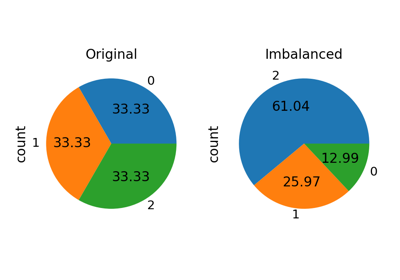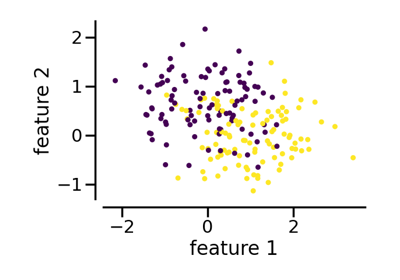# make_imbalance#

imblearn.datasets.make_imbalance(X, y, *, sampling_strategy=None, random_state=None, verbose=False, **kwargs)[source]#

Turn a dataset into an imbalanced dataset with a specific sampling strategy.

A simple toy dataset to visualize clustering and classification algorithms.

Read more in the User Guide.

Parameters
X{array-like, dataframe} of shape (n_samples, n_features)

Matrix containing the data to be imbalanced.

yarray-like of shape (n_samples,)

Corresponding label for each sample in X.

sampling_strategydict or callable,

Ratio to use for resampling the data set.

• When `dict`, the keys correspond to the targeted classes. The values correspond to the desired number of samples for each targeted class.

• When callable, function taking `y` and returns a `dict`. The keys correspond to the targeted classes. The values correspond to the desired number of samples for each class.

random_stateint, RandomState instance or None, default=None

If int, random_state is the seed used by the random number generator; If RandomState instance, random_state is the random number generator; If None, the random number generator is the RandomState instance used by np.random.

verbosebool, default=False

Show information regarding the sampling.

**kwargsdict

Dictionary of additional keyword arguments to pass to `sampling_strategy`.

Returns
X_resampled{ndarray, dataframe} of shape (n_samples_new, n_features)

The array containing the imbalanced data.

y_resampledndarray of shape (n_samples_new)

The corresponding label of `X_resampled`.

Notes

Examples

```>>> from collections import Counter
>>> from imblearn.datasets import make_imbalance
```
```>>> data = load_iris()
>>> X, y = data.data, data.target
>>> print(f'Distribution before imbalancing: {Counter(y)}')
Distribution before imbalancing: Counter({0: 50, 1: 50, 2: 50})
>>> X_res, y_res = make_imbalance(X, y,
...                               sampling_strategy={0: 10, 1: 20, 2: 30},
...                               random_state=42)
>>> print(f'Distribution after imbalancing: {Counter(y_res)}')
Distribution after imbalancing: Counter({2: 30, 1: 20, 0: 10})
```

## Examples using `imblearn.datasets.make_imbalance`#How to use sampling_strategy in imbalanced-learn

How to use ``sampling_strategy`` in imbalanced-learnMulticlass classification with under-sampling

Multiclass classification with under-samplingFitting model on imbalanced datasets and how to fight bias

Fitting model on imbalanced datasets and how to fight biasCreate an imbalanced dataset

Create an imbalanced dataset